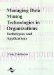# Abstract

 < Day Day Up >

This chapter proposes an approach to the combination of forecasts from a new perspective and uses a new estimation methodology. Concepts from optimization and statistics are combined in order to cast the problem as one of estimating the minimizer of a loss function, which is assumed to be not explicitly known. Using weak assumptions on the unknown loss function, and on the performance distribution of the experts, a density for the expert estimates may be derived. Sufficient conditions are then obtained for reducing the problem to that of statistical mode estimation. By regarding individual forecasts as expert estimates of the minimizer of the decision maker's loss function, the mode of these forecasts becomes a combination forecast. Using the Bayesian approach and the Markov chain Monte Carlo method, an empirical distribution corresponding to the predictive density of the expert estimates can be constructed. Hence the mode can be estimated and a probability interval for the forecast value can also be obtained. The probability interval associated with a combination forecast has not previously been studied in the literature. Thus, the construction of the probability interval is a new contribution. Simulation studies as well as an empirical example are presented to illustrate our method.

 < Day Day Up >Managing Data Mining Technologies in Organizations: Techniques and Applications
ISBN: 1591400570
EAN: 2147483647
Year: 2003
Pages: 174